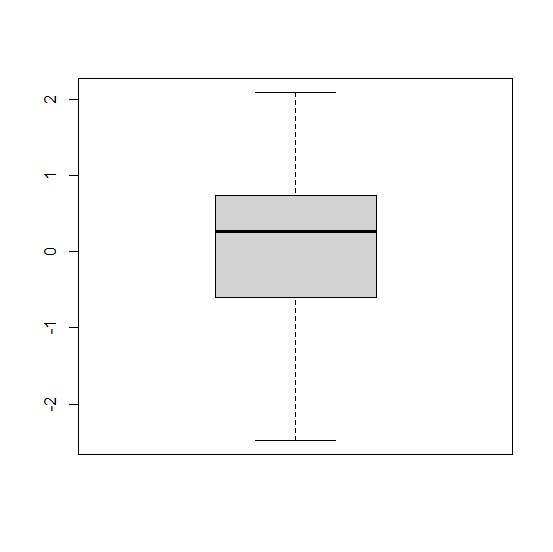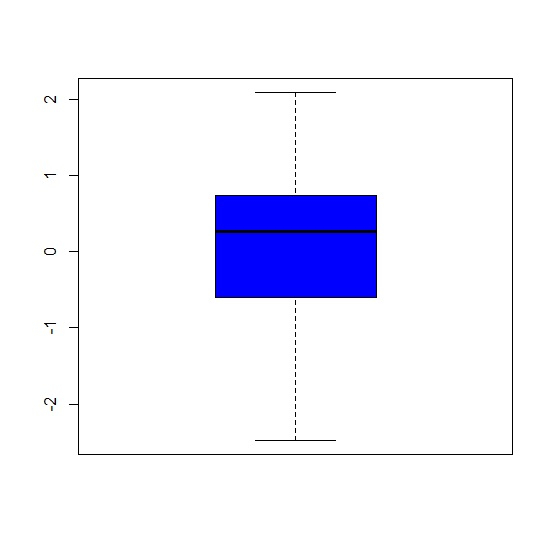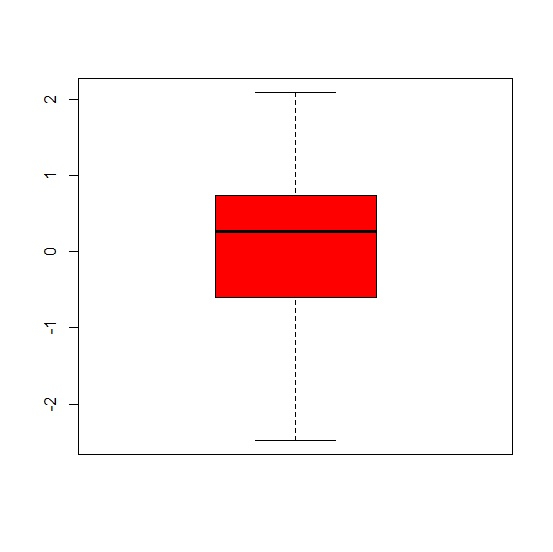# How to change the color of box of boxplot in base R?

To change the color of box of boxplot in base R, we can use col argument inside boxplot function.

For example, if we have a vector called V and we want to create a boxplot of V without red colored box then we can use the following command −

boxplot(x,col="red")

## Example

To change the color of box of boxplot in base R, use the code given below −

x<-rnorm(100)
boxplot(x)

## Output

If you execute the above given code, it generates the following output −To change the color of box of boxplot in base R, use the code given below −

x<-rnorm(100)
boxplot(x,col="blue")

## Outpu

If you execute the above given code, it generates the following output −To change the color of box of boxplot in base R, use the code given below −

x<-rnorm(100)
boxplot(x,col="red")

## Output

If you execute the above given code, it generates the following output −